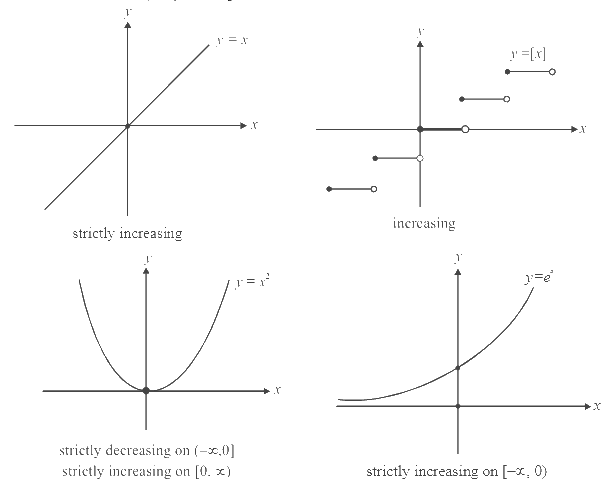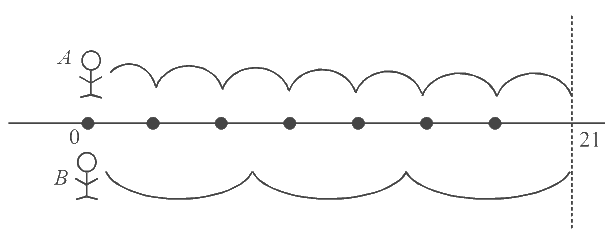# Monotonicity and Periodicity of Functions

Go back to  'Functions'

## INCREASING / DECREASING FUNCTIONS

A function $$f(x)$$  is increasing if  $$f$$  increases as $$x$$  increases.

If  $${x_1} < {x_2}$$    $$\Rightarrow$$      $$f({x_1}) \le f({x_2})$$   $$\Rightarrow$$     function is increasing

$$f({x_1}) < f({x_2})$$   $$\Rightarrow$$       function is strictly increasing

A function $$f(x)$$ is decreasing if $$f$$ decreases as $$x$$ increases.

If   $${x_1} < {x_2}$$   $$\Rightarrow$$   $$f({x_1}) \ge f({x_2})$$    $$\Rightarrow$$  function is decreasing

$$f({x_1}) > f({x_2})$$     $$\Rightarrow$$     function is strictly decreasingIf  $${x_1} < {x_2}$$  and $$f({x_1}) = f({x_2})$$, can $$f$$  be increasing on $$\left[ {{x_1},{x_2}} \right]?$$ Strictly increasing? Decreasing? Strictly decreasing?.

## PERIODIC FUNCTIONS

We have seen in the section on standard functions, that somefunctions, like the trigonometric functions, are repetitive in nature, that is, they repeat after every fixed amount of change in their argument. Such functions are called periodic functions. A periodic function should satisfy

$$f(x + T) = f(x)$$ for every $$x$$              (T is a constant called the period of f).

The smallest such positive value of T is the fundamental period.

For example, $$\text{sin}x$$ has periods $$2\pi, 4\pi, 6\pi, - 4\pi, 2n\pi$$ etc. but the fundamental period is 2$$\pi.$$ Thefunction $$f(x)= \{ x\}$$ is also periodic with a fundamental period of 1. $$f(x) = \tan x$$ is periodic with fundamental period $$\pi,$$ and so on.

Note the following facts about periodic functions:

(a)  If  $$f(x)$$ has period T, then $$f(kx)$$ has a period T/| k |. This is because $$f\left( {k\left( {x + \frac{T}{{|k|}}} \right)} \right) =f(kx \pm T) = f(kx).$$

For example, $$\text{sin }2x$$ has period 2$$\pi$$/2 = $$\pi$$

{$$\pi\,x$$} has a period \begin{align}\frac{1}{\pi }\end{align}

(b)  Consider a function of the form $$z(x) = f(x) \pm g(x) \pm h(x) \pm \ldots \ldots.$$

The fundamental period of $$z(x)$$ would (generally) be the LCM of the individual periods. Why? Suppose two persons A and B start moving fromthe point 0, towards the right, in steps. A’s step is 3 units long. B’s step is 7 units long. At what point will their positions concide? Obviously, LCM (3,7) = 21.The same reasoning applies to the periods of functions

The LCM of the periods of two (or more) function is the first timewhen the two (or more) function start to repeat simultaneously, and hence the combination of these two (or more) functions start to repeat.

For example, $$f(x) = \sin x + \cos x$$ has a period 2$$\pi$$ {LCM (2$$\pi$$, 2$$\pi$$ )}

$$g\left(x \right) = \sin 4x + \cos 6x$$ has a period \begin{align}\pi \{ LCM(\frac{{2\pi}}{4},\frac{{2\pi }}{6}) \equiv \,LCM\left( {\frac{\pi }{2},\frac{\pi }{3}}\right)\} \end{align}

$$h(x) = \left| {sin{\rm{ }}x} \right|$$ has a period $$\pi.$$ (Its easy to see why. Visualise the graph)

$$z{\rm{ }}\left( x \right) = \left| {{\rm{ }}sin{\rm{ }}x{\rm{ }}} \right| + \left| {{\rm{ }}cos{\rm{ }}x{\rm{ }}} \right|{\rm{ }}$$ is, although periodic with period $$\pi$$ {LCM ( $$\pi$$,$$\pi$$ )}, but the fundamental period is\begin{align}\frac{\pi }{2}\end{align}. (Verify). Hence, the LCM rule is not applicable everywhere.

Consider $$y(x) = a|\sin x| + b|\cos x|.$$ Whatis the fundamental period? Think carefully and you will realise that the answer depends upon whether $$a=b$$ or  $$a\ne \, b.$$

If $$a=b,$$ then $$y(x) = a(|\sin x| + |\cos x|)$$ and the fundamental period is \begin{align}\frac{\pi }{2},\end{align} as you just saw. If $$a \, \ne \, b,$$ then the fundamental period is $$\pi.$$

Similarly consider $$v(x) = |\sin nx| + |\cos mx|.$$ Since $$|\text{sin}x |$$ is periodic with $$\pi$$, $$|\text{sin}nx |$$ is periodic with \begin{align}\frac{\pi }{n}\end{align}, $$|\text{cos}mx |$$ is periodic with \begin{align}\frac{\pi }{m}\end{align}. Hence, $$v(x)$$ is periodic with \begin{align}{\rm{LCM\;}}\left( {\frac{\pi }{n},\frac{\pi }{m}} \right)\end{align}.

(Evaluate this LCM for a few examples, like $$m = 2, n = 4 \text{ or } m =3, n = 7$$).

## TRY YOURSELF – V

Q. 1 What are the periods for the followingfunctions?

(a)  $$f(x) = |\sin 2x| + |\sin 3x|$$      (b)   $$f(x) = {\sin ^4}x + {\cos^4}x$$

(c) \begin{align}f(x) = 2\cos \frac{{x - \pi }}{3}\end{align}                (d)   $$f(x) = {\sin ^2}\pi x$$

Q. 2 If $$f$$ is non-periodicand $$g$$ is periodic, what can you say about $$f\left( {g(x)} \right)$$and  $$g\left( {f(x)} \right)$$?

Q. 3 Let $$f$$ be a real-valued function with domain $$\mathbb{R}$$. Now, if for some +ve constant $$‘a’,$$ the equation $$f(x + a) = 1 + {\left\{ {2 - 3f(x) + 3{{\left( {f(x)} \right)}^2} - {{\left( {f(x)} \right)}^3}} \right\}^{1/3}}$$ holds good for all $$x\in \mathbb{R},$$ prove that $$f(x)$$ is a periodic function.

Q. 4 Let $$f$$ be a real valued function defined for $$\mathbb{R}$$, such that for some $$a>0,$$ it satisfies.

\begin{align}f(x + a) = \frac{1}{2} +\sqrt {f(x) - {{\left( {f(x)} \right)}^2}} \end{align}

Prove that $$f(x)$$ is periodic

Q. 5 Let $$f(x,y)$$ be a periodic function satisfying $$f(x,y)= f(2x + 2y,2y - 2x)\forall x,y$$. Define  $$g(x) = f({2^x},0)$$. Show that g is periodic and find its period.

Q. 6 Graph the function $$f(x) = \sqrt {\left\{ {2x}\right\}}$$ and find its period ({.} denotes the fractional part of $$x.$$)